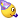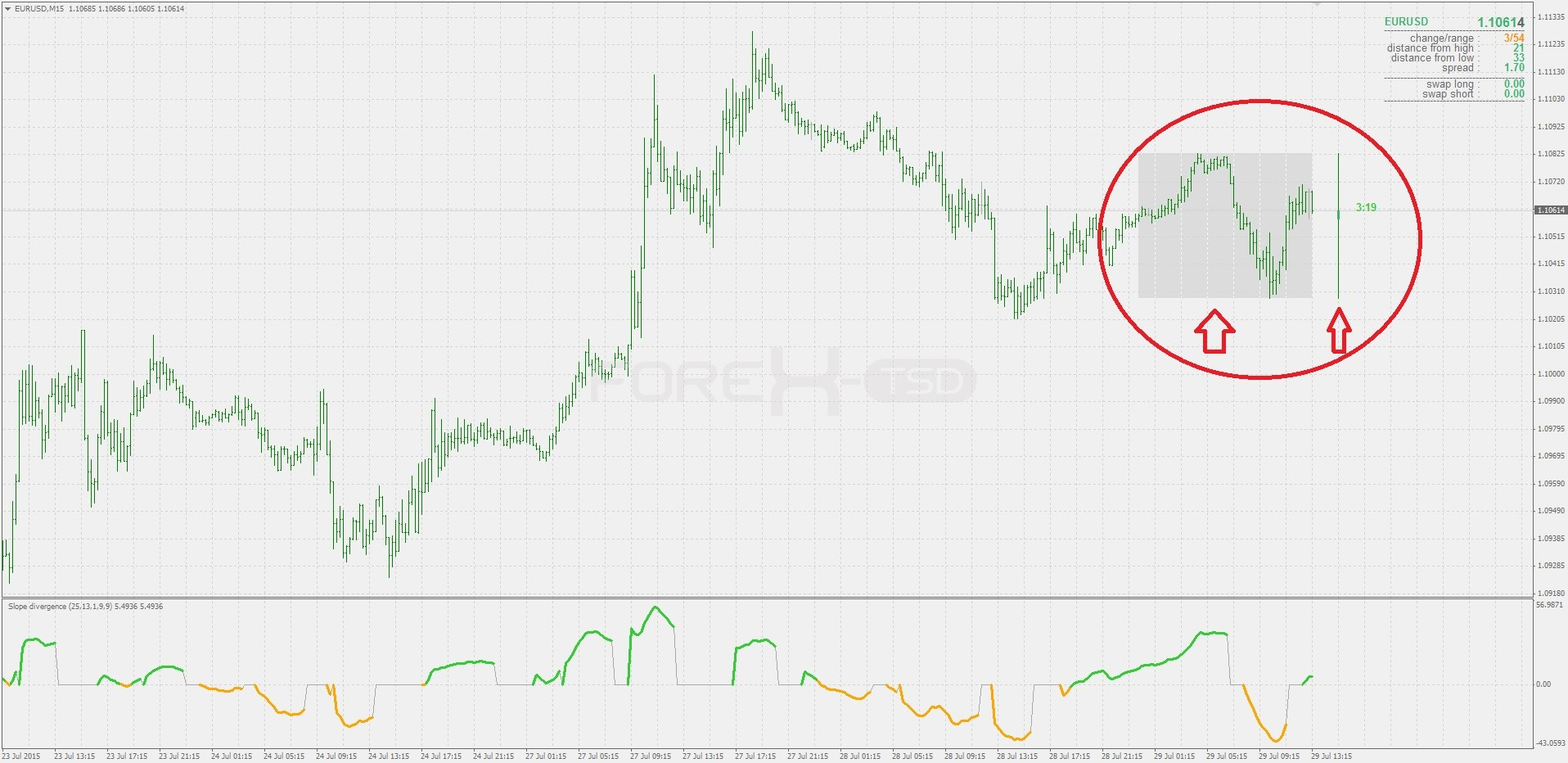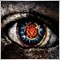# Elite indicators :) - page 979154569

zafmy:Thank you very much for your wonderful work...If I'm not mistaken, I have read regarding above ss, explained by you somewhere which I can't remember.

I hope you can repost here...

thank you

zaf

zaf

that is daily data indicator (from here : https://www.mql5.com/en/forum/178698/page7 )

________________

PS: please re-download the slope divergence RSI - found one issue, which is solved and a corrected indicator is posted at the original post154569

This is a composite TSI (trend strength index) : composite_tsi.ex4

____________________

It allows tsi up to 5th degree calculation. Here is a comparison : upper is the first degree (regular tsi). Middle is the second order tsi and lowest is the third order tsi. As you can see the higher orders are smoother, but it is not the smoothing that is applied to it, but the way of calculating TSI is altered in order to get that kind of results (otherwise we would just add lag). Instead we have almost no lag at all and the smoothing and cycles identification comes at minimal cost967

Thank you malden, I really appreciate

it all work now even posting

Thank you536

Can you change the rsi in this one to adaptive rsi ?stepma_of_rsi.mq4

Files:154569

timmyhanke:
Can you change the rsi in this one to adaptive rsi ?stepma_of_rsi.mq4

timmyhanke

What adaptive rsi are you using?536

timmyhanke What adaptive rsi are you using?

This oneFiles:154569

:
This oneadaptive_rsi.mq4

timmyhanke

That is not an rsi at all. Some more explanation on what exactly it is you can find here : https://www.mql5.com/en/forum/184140

The thing is that rsi expects exact number of bars for calculation (for example you can calculate rsi period 10 but you can not calculate rsi period 10.5 or 9.5) which makes the rsi a "bad candidate" for adapting (that is probably one reason why no too good adaptive rsi was made yet)536

timmyhanke

That is not an rsi at all. Some more explanation on what exactly it is you can find here : https://www.mql5.com/en/forum/184140

The thing is that rsi expects exact number of bars for calculation (for example you can calculate rsi period 10 but you can not calculate rsi period 10.5 or 9.5) which makes the rsi a "bad candidate" for adapting (that is probably one reason why no too good adaptive rsi was made yet)

Ok , But can you change the rsi in the stepma of rsi to an adaptive rsi moving average ?154569

timmyhanke:
Ok , But can you change the rsi in the stepma of rsi to an adaptive rsi moving average ?timmyhankeWhen i wrote to you that i wanted a adaptive rsi crossover with either stepma or moving average i was refering to the indicator that i thought was an adaptive rsi , can you add the crossover to the adaptive rsi moving average ?